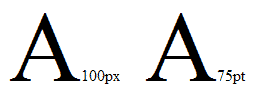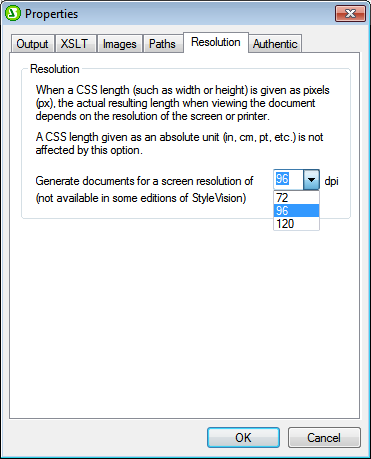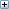# Altova StyleVision 2020 Enterprise Edition

 Pixel Resolution Scroll Home Prev Top Next More

If you use pixels as a unit of length in your SPS, you should be aware that pixel-defined lengths are a function of screen resolution. The corresponding absolute lengths in print could be very different from what you see on screen. In this section, we do the following:

1.Discuss why pixel-defined sizes have two forms: (i) an abstract form, defined in pixels; (ii) an actual size, obtained by resolving the abstract form in terms of a specific screen resolution.

2.Explain StyleVision functionality to deal with this issue.

From pixels to points

A few key points are essential to understanding the factors that affect the abstract and actual dimensions of pixel-defined lengths:

The pixel is a relative unit: its size depends on screen resolution. The higher the resolution, the smaller the pixel. Screen resolution is given with dpi (dots per inch). A dot in the case of screens is a pixel. So, if screen resolution is 72 dpi, then there are 72 pixels (dots) in one inch of a line of pixels. If screen resolution is 96 dpi, then there are 96 pixels in an inch. How many pixels there are in an inch of screen length depends on the screen resolution. The most commonly available screen resolutions today are 72 dpi, 96 dpi, and 120 dpi. The higher the dpi, the smaller will be the pixels.

The length unit known as the point is an absolute unit of length, used most commonly in the printing industry: 72 points make up an inch.

From the above it can be seen that only when screen resolution is 72 dpi will one pixel be equal to one point. For other screen resolutions, the absolute length can be calculated. The table below lists the absolute length (in points) of 100px at various screen resolutions.

 Resolution Pixels Points Factor 72 dpi 100 100 1.00 96 dpi 100 75 0.75 120 dpi 100 60 0.60

To convert pixels to points for each screen resolution, we can use a factor given by the ratio of points in one inch (72) to pixels in one inch (dpi), that is, 72 divided by dpi. The conversion factors for various resolutions are given in the table above. For example: 72 div 96 = 0.75 and 72 div 120 = 0.60. Multiplying the length in pixels by the appropriate conversion factor gives the absolute length in points. So 100px on a 96 dpi screen is 75pt.

Since 72 points make an inch, you can obtain the length in inches by dividing the length in points by 72. For example, 100 points is equal to 100 div 72 inches = 1.389in.

Screen resolution and absolute length

In the previous section we have seen that only when the screen resolution is 72 dpi will the absolute length in points be the same as the number of pixels used to define that length. Screen resolutions on Windows systems, however, are typically not 72 dpi but 96 dpi. This means that the number of points of a pixel-defined length on such a screen will be 75% the number of pixels. For example, in the StyleVision Design View screenshot below, the two characters measure 100px and 75pt, respectively.The reason they are the same height is that the screen resolution in which they appear is 96 dpi. At this resolution 100px is equal to 75pt in absolute terms.

The point to note is that when specifying pixels as a unit of length, be aware of your monitor's screen resolution (normally 96 dpi on Windows systems); it determines the absolute length of the pixel-defined length that you see on screen.

Print output resolution

When an SPS is transformed into a print format, such as PDF, RTF, or Word 2007+, non-absolute pixel lengths must be converted to absolute lengths, such as points or inches (because lengths on paper cannot be defined in terms of pixels). The question is: What factor (or screen resolution) should be used to convert from pixels to points?

In StyleVision, you can select the output dpi for each SPS individually. So, for example, if you select 96 dpi, then a 100px character will be rendered in print output as a 75pt character (see the table above). If you select 72 dpi, then the same character will be rendered in print output as a 100pt character. This system applies to all lengths defined in pixels.

 Note: Conversion to an absolute measure is not carried out for HTML output. For HTML, the original pixel units are passed to the HTML file unchanged.

Setting print output resolution of an SPS

To set the print output resolution of an SPS, click File | Properties. In the Properties dialog that pops up, click the Resolution tab (screenshot below).In the Resolution tab, select the required print resolution. The conversion factor for each listed dpi option is given in the conversion table above (in the section, From Pixels to Points). Multiplying the pixel count by the conversion factor gives the absolute length in points. Note that the conversion to absolute units is applied only to print output formats. The HTML output format will retain the original pixel definitions. Note also that this setting does not change the screen resolution of your monitor.See also